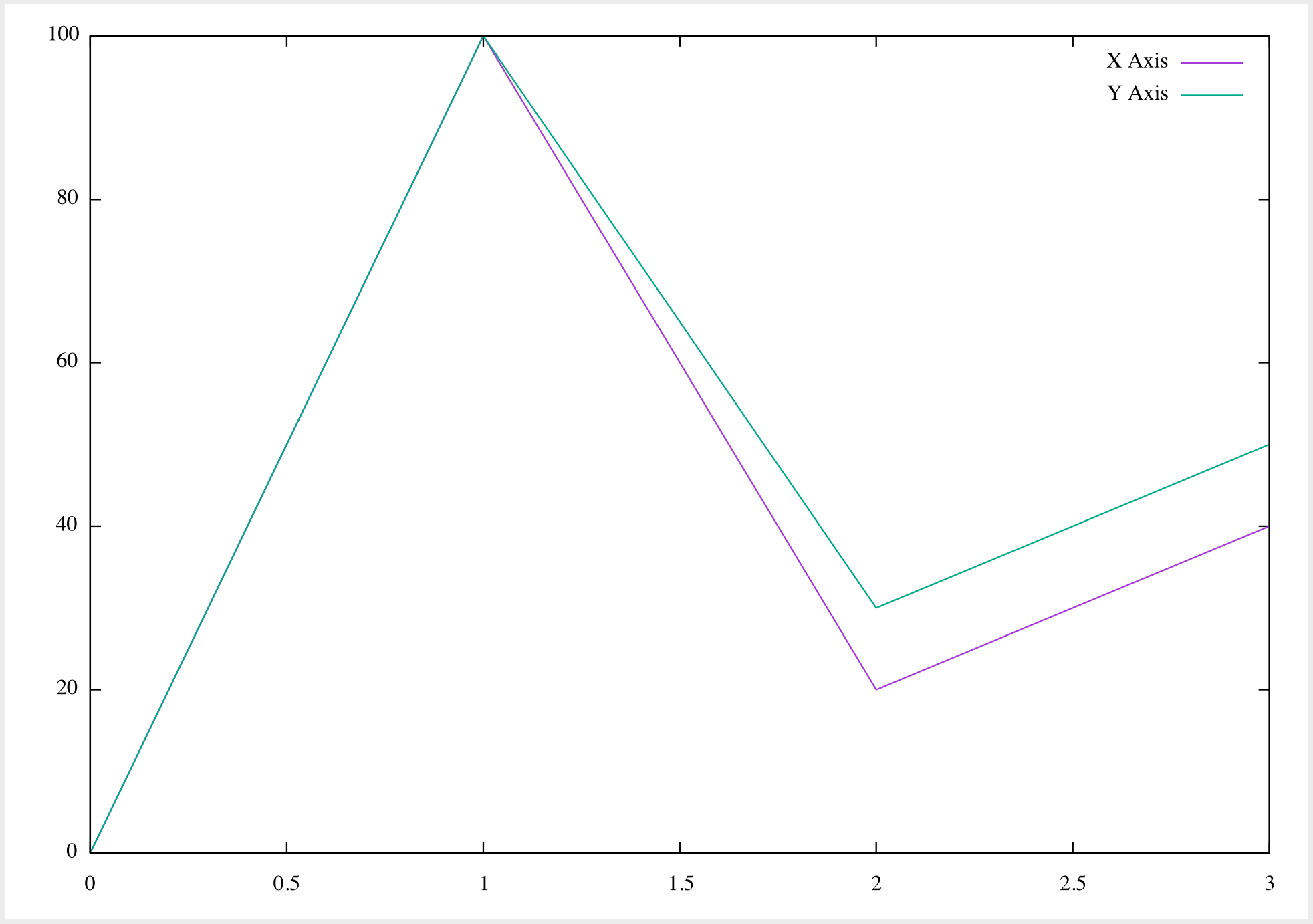I use Emacs as the IDE for most of my projects, one of which required plotting a graph to debug an issue. I have summarised the steps I followed to generate a graph using Emacs, with a mac.

## Prerequisites

2. Install command line tools

``````xcode-select install
``````

``````sudo port install gnuplot +qt5 + x11 +aquaterm +wxwidgets
``````

Refer this setup guide for more details

4. Emacs packages to be included in your .emacs or init.el : `gnuplot`, `gnuplot-mode`

``````(straight-use-package 'gnuplot)
(straight-use-package 'gnuplot-mode)
``````
5. Read the primer for tables in org-mode

## Generating a graph from an org file

1. Create a table with the values you would like to plot

2. Keep your cursor inside the table and invoke the following command

``````M-x org-plot/gnuplot
``````

The short cut key for the same is `C-c " g`

3. The graph shown below will be generated outside the emacs GUI and can be seen in a separate window.4. There are multiple options that can be provided to gnuplot. For instance, in the above plot, since the x-axis was not mentioned in the table it considered both columns to be Y axis. Hence, the two lines - one for each column

5. Options can be provided just above the table you want to plot, in the following format

``````#+PLOT: ind:1
| X Axis | Y Axis |
|--------+--------|
|      0 |      0 |
``````
6. Some options that are useful for simple plots

• ind - `ind` stands for `index` of the column which will act as x-axis. For table 1, we would like our first column to serve as x-axis

``````#+PLOT: ind:1
``````
• with - `with` decides how to plot the graph. By default it uses lines which resulted in the above plot. Here is an example with another value.
``````#+PLOT: ind:1 with:points